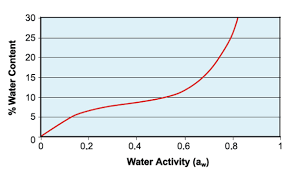## How to Calculate and Solve for Water Activity | Ball Mill SizingThe image above represents water activity.

To compute for water activity, two essential parameters are needed and these parameters are Partial Pressure of Water (Pw) and Equilibrium Vapour Pressure of Water at Same Temperature (V.P.w).

The formula for calculating water activity:

aw = Pw/V.P.w

Where:

aw = Water Activity | Drying
Pw = Partial Pressure of Water
V.P.w = Equilibrium Vapour Pressure of Water at Same Temperature

Let’s solve an example;
Find the water activity when the partial pressure of water is 22 and the equilibrium vapour pressure of water at same temperature is 28.

This implies that;

Pw = Partial Pressure of Water = 22
V.P.w = Equilibrium Vapour Pressure of Water at Same Temperature = 28

aw = Pw/V.P.w
aw = 22/28
aw = 0.78

Therefore, the water activity is 0.78.

Calculating the Partial Pressure of Water when the Water Activity and the Equilibrium Vapour Pressure of Water at Same Temperature is Given.

Pw = aw x V.P.w

Where;

Pw = Partial Pressure of Water
aw = Water Activity | Drying
V.P.w = Equilibrium Vapour Pressure of Water at Same Temperature

Let’s solve an example;
Find the partial pressure of water when the water activity is 24 and the equilibrium vapour pressure of water at same temperature is 14.

This implies that;

aw = Water Activity | Drying = 24
V.P.w = Equilibrium Vapour Pressure of Water at Same Temperature = 14

Pw = aw x V.P.w
Pw = 24 x 14
Pw = 336

Therefore, the partial pressure of water is 336.